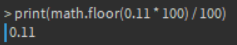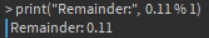# MOD function: x % 1 returns 1?

Hey there, I seem to have encountered a problem.
Theory
Using the MOD function (`%`) returns the remainder of number `a / b`. For example `4 % 5 == 4 / 5 == 0 remainder 4 == 4`. Thus we can conclude that `a % 1` returns the decimal portion of the number. For example, `4.25 % 1 == 0.25`. The result of `x = a % 1` must fall in the range of `0 <= x < 1`, so it is impossible for `x == 1` to evaluate to true.
Issue
The problem, however, is that when I implement this in my code it somehow breaks. I have a number (`0.11`) that I want to floor to 2 decimal places. To do this I use the standard `floor(x * 100) / 100`. For some reason, when I do this to `0.11`, it returns `0.1`. For all other decimals between 0 and 2 it works perfectly (I haven’t tried > 2), but when I try to floor `0.11` it does not work out as it is meant to. I initially assumed this must be some niche bug with the `math.floor` function, so I implemented my own `floor` function like so:

``````function floor(x)
print("Remainder:",  x % 1) -- for testing
return x - (x % 1)
end
``````

This function worked for all cases of `0 <= x < 2` except for `0.11`. The output from the `print` statement for, lets say, `0.1` is `0` (because `0.1 * 100 == 10`, and `10 % 1 == 0`). However, when I try for `0.11` (which should be 0 for the same reason as `0.1` being 0), it prints `Remainder: 1`.

The most peculiar thing is that when I do `print(math.floor(0.11 * 100) / 100)` in the console, it prints `0` instead of 1.

My question is: Why is this happening? And is it avoidable?

For reference, here is the full script:

``````local Player = game.Players.LocalPlayer

local MoneyLabel = script.Parent

local function floor(x)
print("Remainder:", x % 1)
return x - (x % 1)
end

-- MoneyLAbel is a TextLabel, Player.Cash is a NumberValue
MoneyLabel.Text = "\$" .. Player:WaitForChild("Cash").Value
Player.Cash.Changed:Connect(function(new)
print("X:", new, ", Floor'd Value:", floor(new * 100))
MoneyLabel.Text = "\$" .. new
end)
``````

It looks like you’re after some kind of rounding?

``````local function Round(Number)
return print(math.floor(Number + .5))
end

Round(2.50) --> 3
Round(2.49) --> 2
``````

I also recommend using `GetPropertyChangedSignal` in this case.

1 Like

I am not sure what you have managed to do, for me everything seems to be working as expected.

You say that `floor(x * 100) / 100` returns 0.1 however for me it is returning 0.11 as expected.``````0.11 * 100 = 11
math.floor(11) = 11
11/100 = 0.11
``````

You also say that `print("Remainder:", x % 1)` was returning 0 when `x = 0.11`, however for me this was returning `0.11` as expected.You may want to check that your arguments are correct and what you think they should be, it is quite possible that you are modifying the variable somewhere that is causing these weird outputs.

I was after flooring the variable, not rounding, but thank you for your Round function. As for `:GetPropertyChangedSignal`, Instances such as `IntValue` and `StringValue` (and other types of `Value`s) have their own `.Changed` event that overrides the default `Instance.Changed` event and supplies the new value of the `BaseValue` as an argument to the function bound to the event.

Yes, this is why I am absolutely confused. I added print statements to check the values of all vairables at each stage of the process for debugging purposes, however it just doesn’t make sense. When I sequentially work through the algorithm in the console, it works perfectly. I am unsure on how to reproduce this bug in a new environment, becasue it only occurs in that function. The problem is that even `math.floor` is inaccrurate, and using `x % 1` returning 1 is a mathematical imposiblility… yet that is what is returned?

Hi. I’d recommend using IntValues (if you’re using “small” numbers) instead of NumberValues so you don’t fall into floating point errors. Maybe this is what causes the issue…

No, there is no mysterious bug around `math.floor`.It should print 0.11. If it doesn’t, the problem is in the given argument.

You are right, I hadn’t remembered floating point errors by `NumberValues`That might just be the issue.

Even so, this doesn’t explain the fact that when I did `x % 1` I got `1` as a result. Thats an impossiblility by the simple rules of the MOD function: `a % b` gives an answer `x` where `0 <= x < b`, where `b` is the upper bound and is not included in the range.